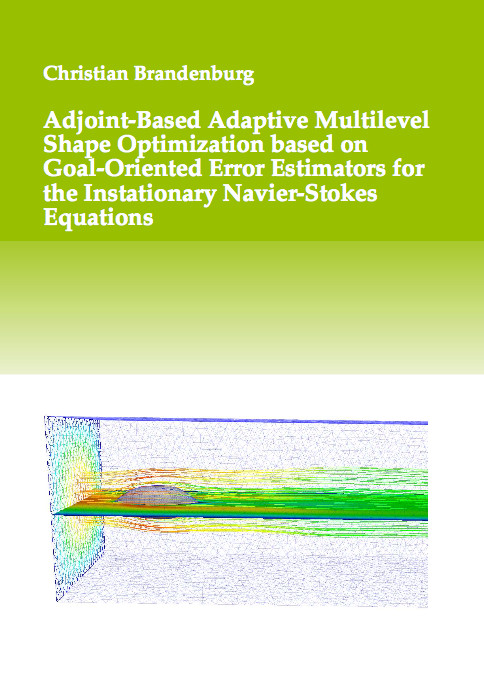Datenbestand vom 10. Dezember 2019aktualisiert am 10. Dezember 2019

# ISBN 978-3-8439-0076-8Euro 84,00 inkl. 7% MwSt

978-3-8439-0076-8, Reihe Mathematik

Christian Brandenburg
Adjoint-Based Adaptive Multilevel Shape Optimization based on Goal-Oriented Error Estimators for the Instationary Navier-Stokes Equations

221 Seiten, Dissertation Technische Universität Darmstadt (2011), Softcover, B5

## Zusammenfassung / Abstract

We have presented an approach to shape optimization that is based on transformations to a reference domain. This approach addresses the main questions concerning shape optimization problems, that is the existence of optimal solutions, the derivation of optimality conditions and the computation of shape derivatives, as well as the numerical computation of optimal solutions, in a consistent way. The transformation approach was illustrated using the instationary heat equation, for which we obtained existence results for optimal solutions and first order necessary optimality conditions that form the basis for the computation of shape derivatives. Afterwards, we have transfered these results to shape optimization problems governed by the instationary Navier-Stokes equations.

To numerically solve shape optimization problems, we use finite element discretizations of the infinite dimensional problem. For solving the discrete problems efficiently, we use an adaptive multilevel shape optimization procedure where the adaptivity is based on goal-oriented error estimators. In particular, we extend known results for goal-oriented error estimators in the context of optimal control problems to the situation at hand.

Finally, we have presented numerical results to demonstrate that the transformation approach is indeed well suited to solve shape optimization problems numerically. Using adaptivity based on goal-oriented error estimators, we are able to obtain optimal solutions to drag optimization problems efficiently by performing a significant part of the optimization iterations on coarse discretizations.